Developer guides / KerasCV / Classification with KerasCV

# Classification with KerasCV

Author: lukewood
Date created: 03/28/2023
Description: Use KerasCV to train powerful image classifiers.View in ColabGitHub source

Classification is the process of predicting a categorical label for a given input image. While classification is a relatively straightforward computer vision task, modern approaches still are built of several complex components. Luckily, KerasCV provides APIs to construct commonly used components.

This guide demonstrates KerasCV's modular approach to solving image classification problems at three levels of complexity:

• Inference with a pretrained classifier
• Fine-tuning a pretrained backbone
• Training a image classifier from scratch

KerasCV uses Keras 3 to work with any of TensorFlow, PyTorch or Jax. In the guide below, we will use the `jax` backend. This guide runs in TensorFlow or PyTorch backends with zero changes, simply update the `KERAS_BACKEND` below.

We use Professor Keras, the official Keras mascot, as a visual reference for the complexity of the material: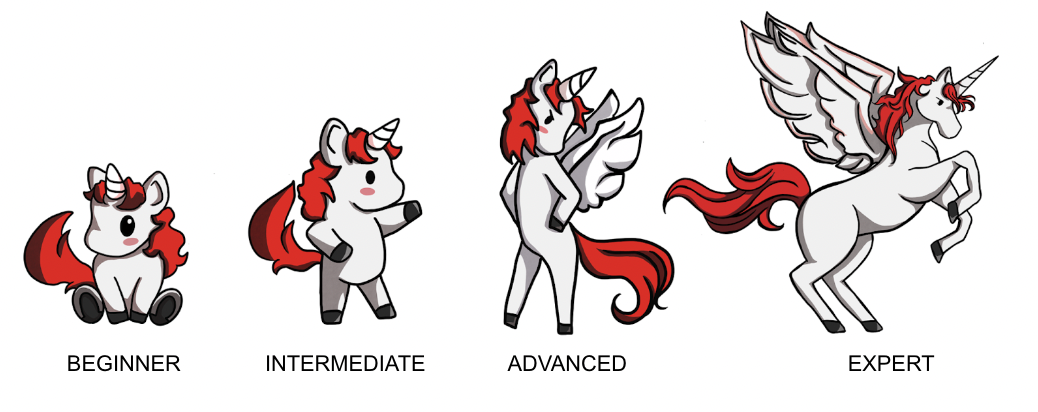``````!pip install -q —upgrade keras-cv
``````
``````import os

os.environ["KERAS_BACKEND"] = "jax"  # @param ["tensorflow", "jax", "torch"]

import json
import math
import numpy as np

import keras
from keras import losses
from keras import ops
from keras import optimizers
from keras.optimizers import schedules
from keras import metrics

import keras_cv

# Import tensorflow for [`tf.data`](https://www.tensorflow.org/api_docs/python/tf/data) and its preprocessing functions
import tensorflow as tf
import tensorflow_datasets as tfds
``````

## Inference with a pretrained classifier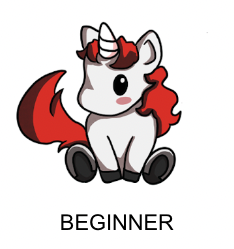Let's get started with the simplest KerasCV API: a pretrained classifier. In this example, we will construct a classifier that was pretrained on the ImageNet dataset. We'll use this model to solve the age old "Cat or Dog" problem.

The highest level module in KerasCV is a task. A task is a `keras.Model` consisting of a (generally pretrained) backbone model and task-specific layers. Here's an example using `keras_cv.models.ImageClassifier` with an EfficientNetV2B0 Backbone.

EfficientNetV2B0 is a great starting model when constructing an image classification pipeline. This architecture manages to achieve high accuracy, while using a parameter count of 7M. If an EfficientNetV2B0 is not powerful enough for the task you are hoping to solve, be sure to check out KerasCV's other available Backbones!

``````classifier = keras_cv.models.ImageClassifier.from_preset(
"efficientnetv2_b0_imagenet_classifier"
)
``````

You may notice a small deviation from the old `keras.applications` API; where you would construct the class with `EfficientNetV2B0(weights="imagenet")`. While the old API was great for classification, it did not scale effectively to other use cases that required complex architectures, like object deteciton and semantic segmentation.

Now that our classifier is built, let's apply it to this cute cat picture!

``````filepath = keras.utils.get_file(origin="https://i.imgur.com/9i63gLN.jpg")
image = np.array(image)
keras_cv.visualization.plot_image_gallery(
np.array([image]), rows=1, cols=1, value_range=(0, 255), show=True, scale=4
)
``````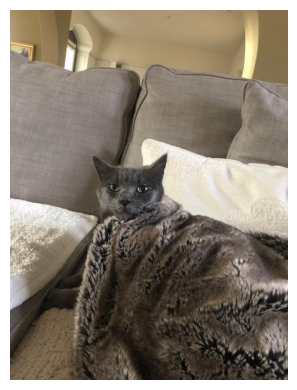Next, let's get some predictions from our classifier:

``````predictions = classifier.predict(np.expand_dims(image, axis=0))
``````
`````` 1/1 ━━━━━━━━━━━━━━━━━━━━ 4s 4s/step
``````

Predictions come in the form of softmax-ed category rankings. We can find the index of the top classes using a simple argsort function:

``````top_classes = predictions.argsort(axis=-1)
``````

In order to decode the class mappings, we can construct a mapping from category indices to ImageNet class names. For convenience, I've stored the ImageNet class mapping in a GitHub gist. Let's download and load it now.

``````classes = keras.utils.get_file(
origin="https://gist.githubusercontent.com/LukeWood/62eebcd5c5c4a4d0e0b7845780f76d55/raw/fde63e5e4c09e2fa0a3436680f436bdcb8325aac/ImagenetClassnames.json"
)
with open(classes, "rb") as f:
``````
``````Downloading data from https://gist.githubusercontent.com/LukeWood/62eebcd5c5c4a4d0e0b7845780f76d55/raw/fde63e5e4c09e2fa0a3436680f436bdcb8325aac/ImagenetClassnames.json
33567/33567 ━━━━━━━━━━━━━━━━━━━━ 0s 0us/step
``````

Now we can simply look up the class names via index:

``````top_two = [classes[str(i)] for i in top_classes[-2:]]
print("Top two classes are:", top_two)
``````
``````Top two classes are: ['Egyptian cat', 'velvet']
``````

Great! Both of these appear to be correct! However, one of the classes is "Velvet". We're trying to classify Cats VS Dogs. We don't care about the velvet blanket!

Ideally, we'd have a classifier that only performs computation to determine if an image is a cat or a dog, and has all of its resources dedicated to this task. This can be solved by fine tuning our own classifier.

# Fine tuning a pretrained classifier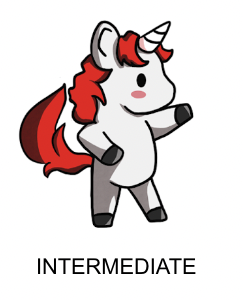When labeled images specific to our task are available, fine-tuning a custom classifier can improve performance. If we want to train a Cats vs Dogs Classifier, using explicitly labeled Cat vs Dog data should perform better than the generic classifier! For many tasks, no relevant pretrained model will be available (e.g., categorizing images specific to your application).

``````BATCH_SIZE = 32
IMAGE_SIZE = (224, 224)
AUTOTUNE = tf.data.AUTOTUNE
tfds.disable_progress_bar()

data, dataset_info = tfds.load("cats_vs_dogs", with_info=True, as_supervised=True)
train_steps_per_epoch = dataset_info.splits["train"].num_examples // BATCH_SIZE
train_dataset = data["train"]

num_classes = dataset_info.features["label"].num_classes

resizing = keras_cv.layers.Resizing(
IMAGE_SIZE, IMAGE_SIZE, crop_to_aspect_ratio=True
)

def preprocess_inputs(image, label):
image = tf.cast(image, tf.float32)
# Staticly resize images as we only iterate the dataset once.
return resizing(image), tf.one_hot(label, num_classes)

# Shuffle the dataset to increase diversity of batches.
# 10*BATCH_SIZE follows the assumption that bigger machines can handle bigger
# shuffle buffers.
train_dataset = train_dataset.shuffle(
10 * BATCH_SIZE, reshuffle_each_iteration=True
).map(preprocess_inputs, num_parallel_calls=AUTOTUNE)
train_dataset = train_dataset.batch(BATCH_SIZE)

images = next(iter(train_dataset.take(1)))
keras_cv.visualization.plot_image_gallery(images, value_range=(0, 255))
``````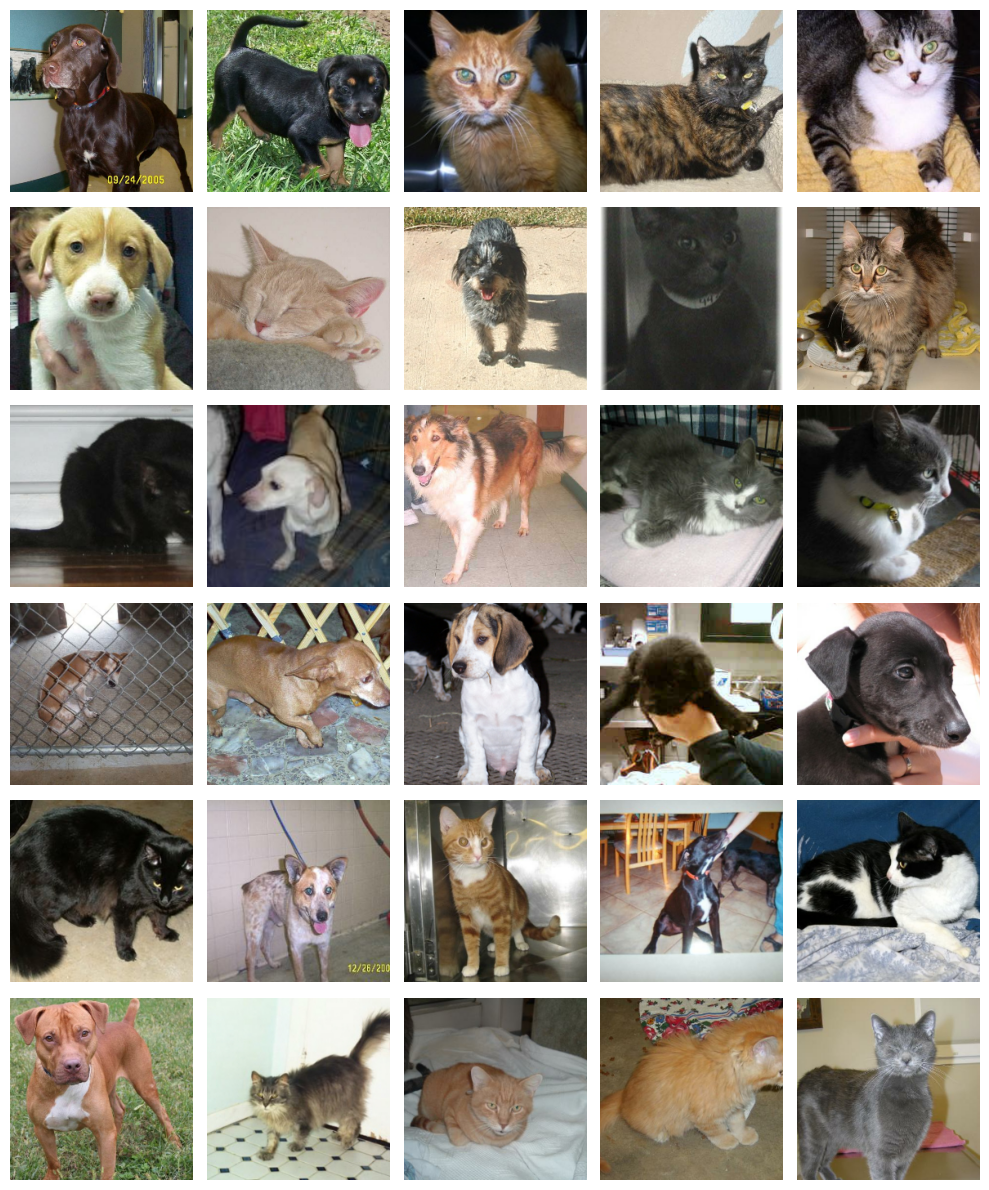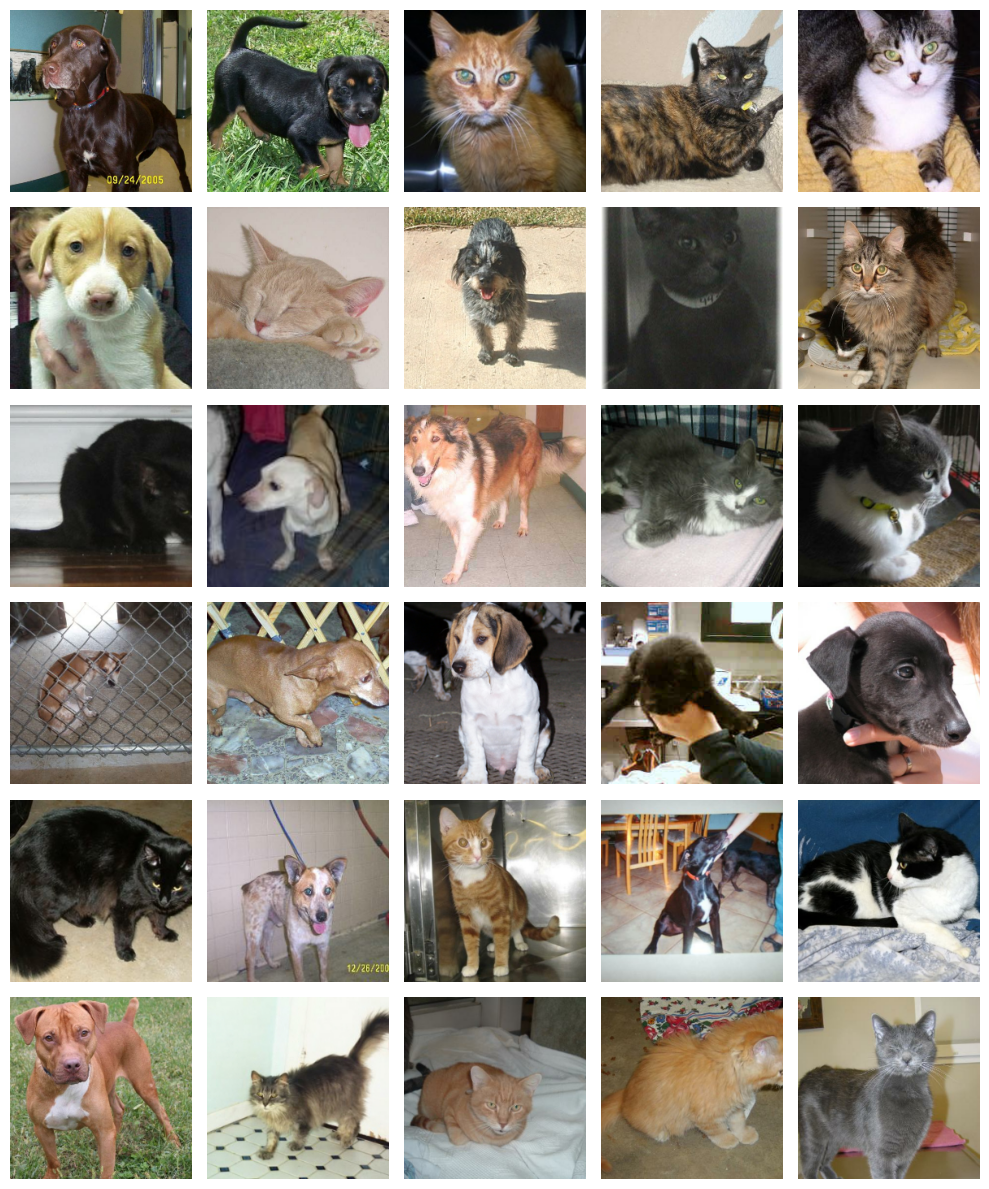Meow!

Next let's construct our model. The use of imagenet in the preset name indicates that the backbone was pretrained on the ImageNet dataset. Pretrained backbones extract more information from our labeled examples by leveraging patterns extracted from potentially much larger datasets.

Next lets put together our classifier:

``````model = keras_cv.models.ImageClassifier.from_preset(
"efficientnetv2_b0_imagenet", num_classes=2
)
model.compile(
loss="categorical_crossentropy",
optimizer=keras.optimizers.SGD(learning_rate=0.01),
metrics=["accuracy"],
)
``````
``````Downloading data from https://storage.googleapis.com/keras-cv/models/efficientnetv2b0/imagenet/classification-v0-notop.h5
24029184/24029184 ━━━━━━━━━━━━━━━━━━━━ 1s 0us/step
``````

Here our classifier is just a simple `keras.Sequential`. All that is left to do is call `model.fit()`:

``````model.fit(train_dataset)
``````
`````` 216/727 ━━━━━[37m━━━━━━━━━━━━━━━  15s 30ms/step - accuracy: 0.8433 - loss: 0.5113

Corrupt JPEG data: 99 extraneous bytes before marker 0xd9

254/727 ━━━━━━[37m━━━━━━━━━━━━━━  14s 30ms/step - accuracy: 0.8535 - loss: 0.4941

Warning: unknown JFIF revision number 0.00

266/727 ━━━━━━━[37m━━━━━━━━━━━━━  14s 30ms/step - accuracy: 0.8563 - loss: 0.4891

Corrupt JPEG data: 396 extraneous bytes before marker 0xd9

310/727 ━━━━━━━━[37m━━━━━━━━━━━━  12s 30ms/step - accuracy: 0.8651 - loss: 0.4719

Corrupt JPEG data: 162 extraneous bytes before marker 0xd9

358/727 ━━━━━━━━━[37m━━━━━━━━━━━  11s 30ms/step - accuracy: 0.8729 - loss: 0.4550

Corrupt JPEG data: 252 extraneous bytes before marker 0xd9
Corrupt JPEG data: 65 extraneous bytes before marker 0xd9

374/727 ━━━━━━━━━━[37m━━━━━━━━━━  10s 30ms/step - accuracy: 0.8752 - loss: 0.4497

Corrupt JPEG data: 1403 extraneous bytes before marker 0xd9

534/727 ━━━━━━━━━━━━━━[37m━━━━━━  5s 30ms/step - accuracy: 0.8921 - loss: 0.4056

Corrupt JPEG data: 214 extraneous bytes before marker 0xd9

636/727 ━━━━━━━━━━━━━━━━━[37m━━━  2s 30ms/step - accuracy: 0.8993 - loss: 0.3837

Corrupt JPEG data: 2226 extraneous bytes before marker 0xd9

654/727 ━━━━━━━━━━━━━━━━━[37m━━━  2s 30ms/step - accuracy: 0.9004 - loss: 0.3802

Corrupt JPEG data: 128 extraneous bytes before marker 0xd9

668/727 ━━━━━━━━━━━━━━━━━━[37m━━  1s 30ms/step - accuracy: 0.9012 - loss: 0.3775

Corrupt JPEG data: 239 extraneous bytes before marker 0xd9

704/727 ━━━━━━━━━━━━━━━━━━━[37m━  0s 30ms/step - accuracy: 0.9032 - loss: 0.3709

Corrupt JPEG data: 1153 extraneous bytes before marker 0xd9

712/727 ━━━━━━━━━━━━━━━━━━━[37m━  0s 30ms/step - accuracy: 0.9036 - loss: 0.3695

Corrupt JPEG data: 228 extraneous bytes before marker 0xd9

727/727 ━━━━━━━━━━━━━━━━━━━━ 69s 62ms/step - accuracy: 0.9045 - loss: 0.3667

<keras.src.callbacks.history.History at 0x7fce380df100>
``````

Let's look at how our model performs after the fine tuning:

``````predictions = model.predict(np.expand_dims(image, axis=0))

classes = {0: "cat", 1: "dog"}
print("Top class is:", classes[predictions.argmax()])
``````
`````` 1/1 ━━━━━━━━━━━━━━━━━━━━ 3s 3s/step
Top class is: cat
``````

Awesome - looks like the model correctly classified the image.

# Train a Classifier from Scratch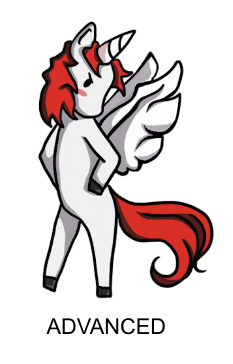Now that we've gotten our hands dirty with classification, let's take on one last task: training a classification model from scratch! A standard benchmark for image classification is the ImageNet dataset, however due to licensing constraints we will use the CalTech 101 image classification dataset in this tutorial. While we use the simpler CalTech 101 dataset in this guide, the same training template may be used on ImageNet to achieve near state-of-the-art scores.

``````NUM_CLASSES = 101
# Change epochs to 100~ to fully train.
EPOCHS = 1

def package_inputs(image, label):
return {"images": image, "labels": tf.one_hot(label, NUM_CLASSES)}

"caltech101", split=["train", "test"], as_supervised="true"
)
train_ds = train_ds.map(package_inputs, num_parallel_calls=tf.data.AUTOTUNE)
eval_ds = eval_ds.map(package_inputs, num_parallel_calls=tf.data.AUTOTUNE)

train_ds = train_ds.shuffle(BATCH_SIZE * 16)
``````
`````` Downloading and preparing dataset 125.64 MiB (download: 125.64 MiB, generated: 132.86 MiB, total: 258.50 MiB) to /usr/local/google/home/rameshsampath/tensorflow_datasets/caltech101/3.0.1...
``````

The CalTech101 dataset has different sizes for every image, so we use the `ragged_batch()` API to batch them together while maintaining each individual image's shape information.

``````train_ds = train_ds.ragged_batch(BATCH_SIZE)
eval_ds = eval_ds.ragged_batch(BATCH_SIZE)

batch = next(iter(train_ds.take(1)))
image_batch = batch["images"]
label_batch = batch["labels"]

keras_cv.visualization.plot_image_gallery(
image_batch.to_tensor(),
rows=3,
cols=3,
value_range=(0, 255),
show=True,
)
``````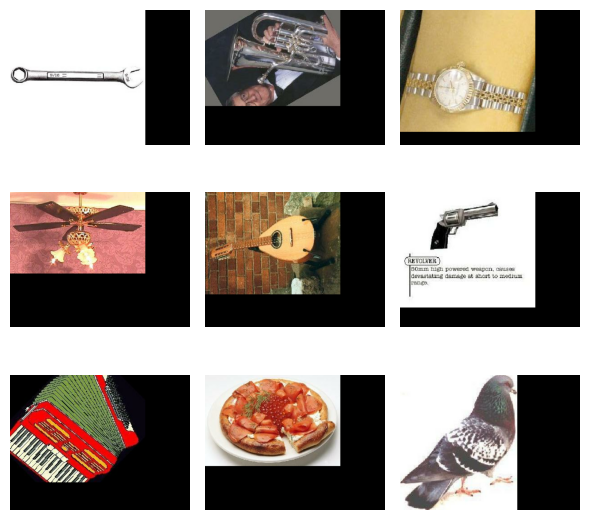## Data Augmentation

In our previous finetuning exmaple, we performed a static resizing operation and did not utilize any image augmentation. This is because a single pass over the training set was sufficient to achieve decent results. When training to solve a more difficult task, you'll want to include data augmentation in your data pipeline.

Data augmentation is a technique to make your model robust to changes in input data such as lighting, cropping, and orientation. KerasCV includes some of the most useful augmentations in the `keras_cv.layers` API. Creating an optimal pipeline of augmentations is an art, but in this section of the guide we'll offer some tips on best practices for classification.

One caveat to be aware of with image data augmentation is that you must be careful to not shift your augmented data distribution too far from the original data distribution. The goal is to prevent overfitting and increase generalization, but samples that lie completely out of the data distribution simply add noise to the training process.

The first augmentation we'll use is `RandomFlip`. This augmentation behaves more or less how you'd expect: it either flips the image or not. While this augmentation is useful in CalTech101 and ImageNet, it should be noted that it should not be used on tasks where the data distribution is not vertical mirror invariant. An example of a dataset where this occurs is MNIST hand written digits. Flipping a `6` over the vertical axis will make the digit appear more like a `7` than a `6`, but the label will still show a `6`.

``````random_flip = keras_cv.layers.RandomFlip()
augmenters = [random_flip]

image_batch = random_flip(image_batch)
keras_cv.visualization.plot_image_gallery(
image_batch.to_tensor(),
rows=3,
cols=3,
value_range=(0, 255),
show=True,
)
``````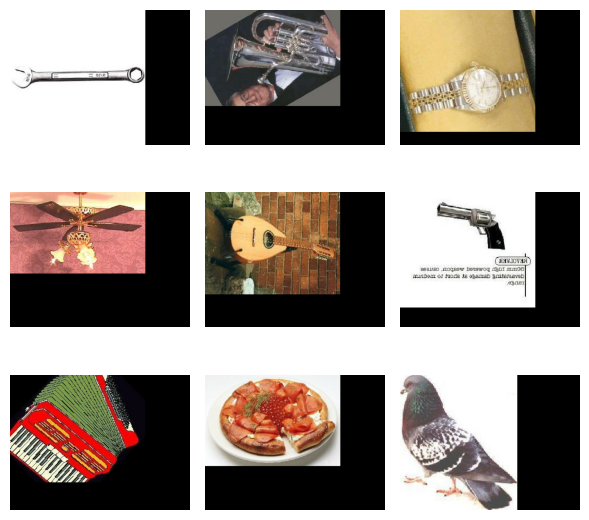Half of the images have been flipped!

The next augmentation we'll use is `RandomCropAndResize`. This operation selects a random subset of the image, then resizes it to the provided target size. By using this augmentation, we force our classifier to become spatially invariant. Additionally, this layer accepts an `aspect_ratio_factor` which can be used to distort the aspect ratio of the image. While this can improve model performance, it should be used with caution. It is very easy for an aspect ratio distortion to shift a sample too far from the original training set's data distribution. Remember - the goal of data augmentation is to produce more training samples that align with the data distribution of your training set!

`RandomCropAndResize` also can handle `tf.RaggedTensor` inputs. In the CalTech101 image dataset images come in a wide variety of sizes. As such they cannot easily be batched together into a dense training batch. Luckily, `RandomCropAndResize` handles the Ragged -> Dense conversion process for you!

Let's add a `RandomCropAndResize` to our set of augmentations:

``````crop_and_resize = keras_cv.layers.RandomCropAndResize(
target_size=IMAGE_SIZE,
crop_area_factor=(0.8, 1.0),
aspect_ratio_factor=(0.9, 1.1),
)
augmenters += [crop_and_resize]

image_batch = crop_and_resize(image_batch)
keras_cv.visualization.plot_image_gallery(
image_batch,
rows=3,
cols=3,
value_range=(0, 255),
show=True,
)
``````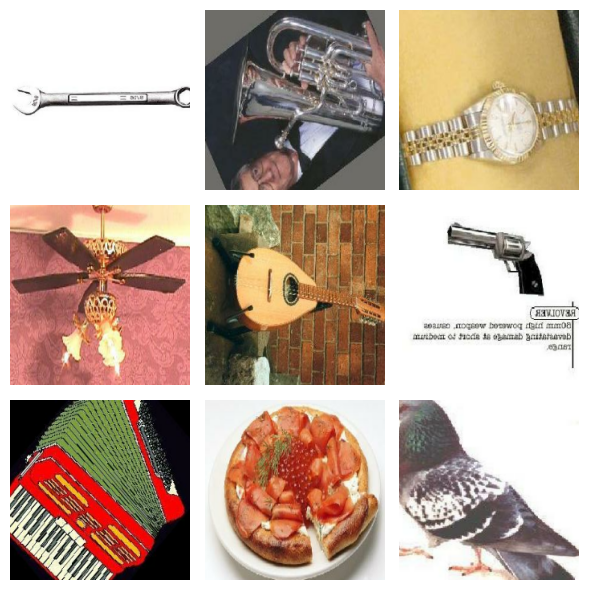Great! We are now working with a batch of dense images. Next up, lets include some spatial and color-based jitter to our training set. This will allow us to produce a classifier that is robust to lighting flickers, shadows, and more.

There are limitless ways to augment an image by altering color and spatial features, but perhaps the most battle tested technique is `RandAugment`. `RandAugment` is actually a set of 10 different augmentations: `AutoContrast`, `Equalize`, `Solarize`, `RandomColorJitter`, `RandomContrast`, `RandomBrightness`, `ShearX`, `ShearY`, `TranslateX` and `TranslateY`. At inference time, `num_augmentations` augmenters are sampled for each image, and random magnitude factors are sampled for each. These augmentations are then applied sequentially.

KerasCV makes tuning these parameters easy using the `augmentations_per_image` and `magnitude` parameters! Let's take it for a spin:

``````rand_augment = keras_cv.layers.RandAugment(
augmentations_per_image=3,
value_range=(0, 255),
magnitude=0.3,
magnitude_stddev=0.2,
rate=1.0,
)
augmenters += [rand_augment]

image_batch = rand_augment(image_batch)
keras_cv.visualization.plot_image_gallery(
image_batch,
rows=3,
cols=3,
value_range=(0, 255),
show=True,
)
``````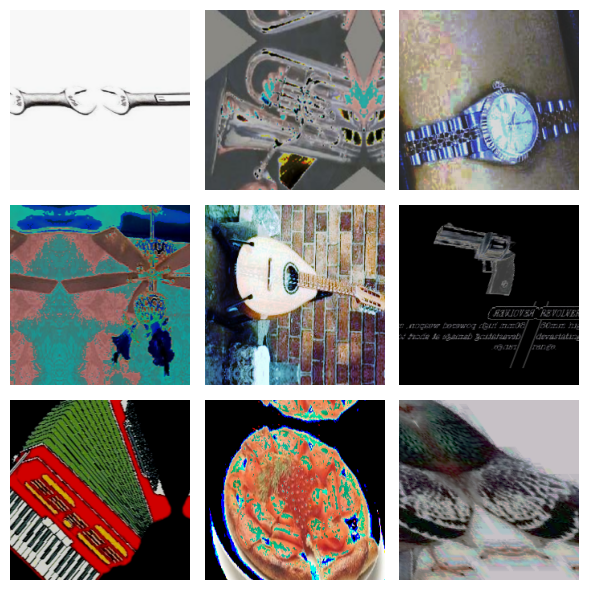Looks great; but we're not done yet! What if an image is missing one critical feature of a class? For example, what if a leaf is blocking the view of a cat's ear, but our classifier learned to classify cats simply by observing their ears?

One easy approach to tackling this is to use `RandomCutout`, which randomly strips out a sub-section of the image:

``````random_cutout = keras_cv.layers.RandomCutout(width_factor=0.4, height_factor=0.4)
keras_cv.visualization.plot_image_gallery(
random_cutout(image_batch),
rows=3,
cols=3,
value_range=(0, 255),
show=True,
)
``````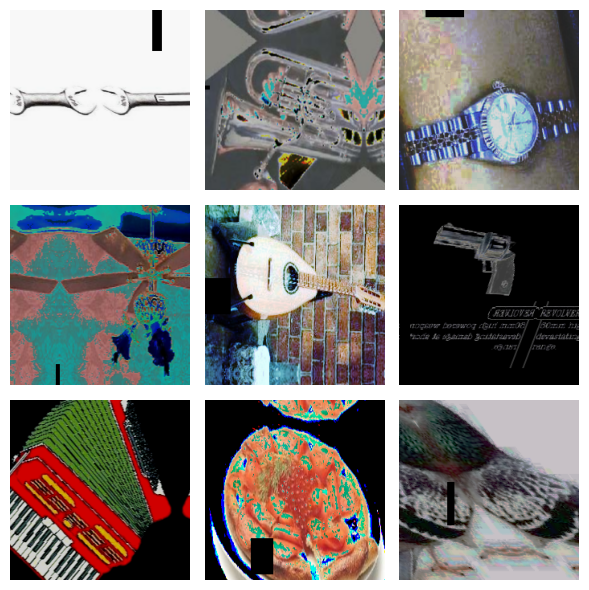While this tackles the problem reasonably well, it can cause the classifier to develop responses to borders between features and black pixel areas caused by the cutout.

`CutMix` solves the same issue by using a more complex (and more effective) technique. Instead of replacing the cut-out areas with black pixels, `CutMix` replaces these regions with regions of other images sampled from within your training set! Following this replacement, the image's classification label is updated to be a blend of the original and mixed image's class label.

What does this look like in practice? Let's check it out:

``````cut_mix = keras_cv.layers.CutMix()
# CutMix needs to modify both images and labels
inputs = {"images": image_batch, "labels": label_batch}

keras_cv.visualization.plot_image_gallery(
cut_mix(inputs)["images"],
rows=3,
cols=3,
value_range=(0, 255),
show=True,
)
``````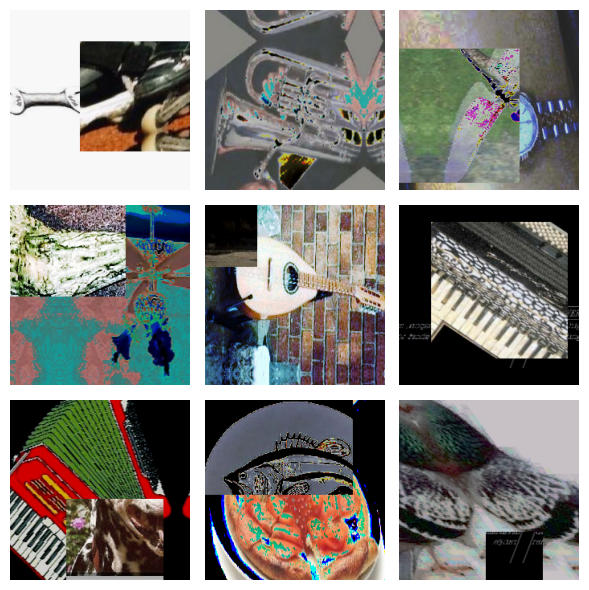Let's hold off from adding it to our augmenter for a minute - more on that soon!

Next, let's look into `MixUp()`. Unfortunately, while `MixUp()` has been empirically shown to substantially improve both the robustness and the generalization of the trained model, it is not well-understood why such improvement occurs... but a little alchemy never hurt anyone!

`MixUp()` works by sampling two images from a batch, then proceeding to literally blend together their pixel intensities as well as their classification labels.

Let's see it in action:

``````mix_up = keras_cv.layers.MixUp()
# MixUp needs to modify both images and labels
inputs = {"images": image_batch, "labels": label_batch}

keras_cv.visualization.plot_image_gallery(
mix_up(inputs)["images"],
rows=3,
cols=3,
value_range=(0, 255),
show=True,
)
``````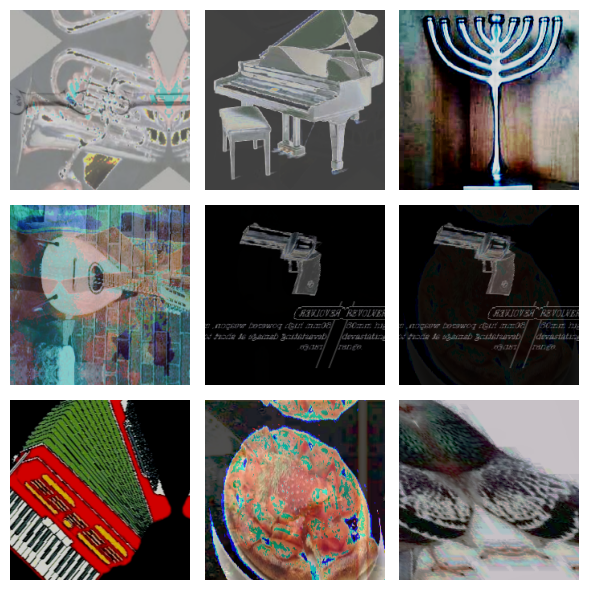If you look closely, you'll see that the images have been blended together.

Instead of applying `CutMix()` and `MixUp()` to every image, we instead pick one or the other to apply to each batch. This can be expressed using `keras_cv.layers.RandomChoice()`

``````cut_mix_or_mix_up = keras_cv.layers.RandomChoice([cut_mix, mix_up], batchwise=True)
augmenters += [cut_mix_or_mix_up]
``````

Now let's apply our final augmenter to the training data:

``````def create_augmenter_fn(augmenters):
def augmenter_fn(inputs):
for augmenter in augmenters:
inputs = augmenter(inputs)
return inputs

return augmenter_fn

augmenter_fn = create_augmenter_fn(augmenters)
train_ds = train_ds.map(augmenter_fn, num_parallel_calls=tf.data.AUTOTUNE)

image_batch = next(iter(train_ds.take(1)))["images"]
keras_cv.visualization.plot_image_gallery(
image_batch,
rows=3,
cols=3,
value_range=(0, 255),
show=True,
)
``````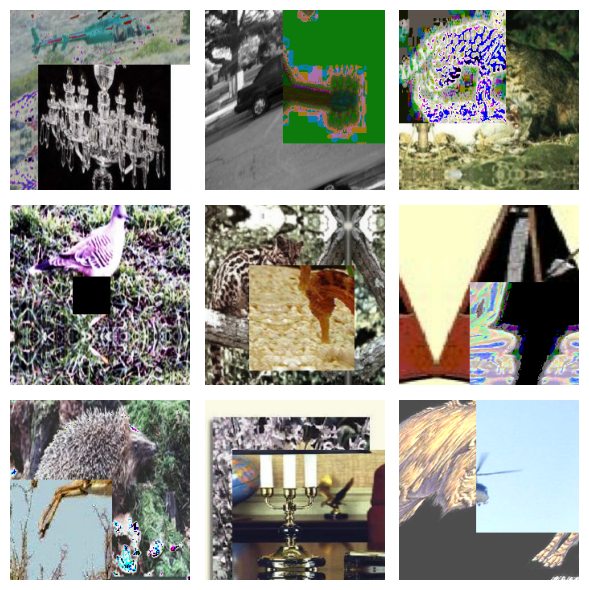We also need to resize our evaluation set to get dense batches of the image size expected by our model. We use the deterministic `keras_cv.layers.Resizing` in this case to avoid adding noise to our evaluation metric.

``````inference_resizing = keras_cv.layers.Resizing(
IMAGE_SIZE, IMAGE_SIZE, crop_to_aspect_ratio=True
)
eval_ds = eval_ds.map(inference_resizing, num_parallel_calls=tf.data.AUTOTUNE)

inference_resizing = keras_cv.layers.Resizing(
IMAGE_SIZE, IMAGE_SIZE, crop_to_aspect_ratio=True
)
eval_ds = eval_ds.map(inference_resizing, num_parallel_calls=tf.data.AUTOTUNE)

image_batch = next(iter(eval_ds.take(1)))["images"]
keras_cv.visualization.plot_image_gallery(
image_batch,
rows=3,
cols=3,
value_range=(0, 255),
show=True,
)
``````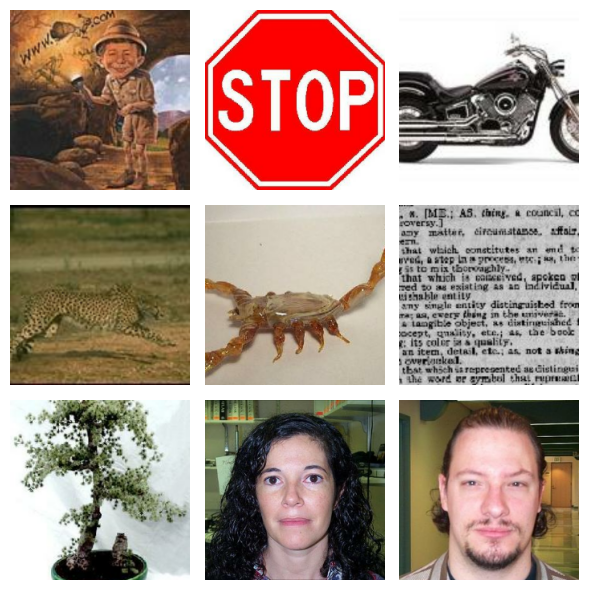Finally, lets unpackage our datasets and prepare to pass them to `model.fit()`, which accepts a tuple of `(images, labels)`.

``````def unpackage_dict(inputs):
return inputs["images"], inputs["labels"]

train_ds = train_ds.map(unpackage_dict, num_parallel_calls=tf.data.AUTOTUNE)
eval_ds = eval_ds.map(unpackage_dict, num_parallel_calls=tf.data.AUTOTUNE)
``````

Data augmentation is by far the hardest piece of training a modern classifier. Congratulations on making it this far!

## Optimizer Tuning

To achieve optimal performance, we need to use a learning rate schedule instead of a single learning rate. While we won't go into detail on the Cosine decay with warmup schedule used here, you can read more about it here.

``````def lr_warmup_cosine_decay(
global_step,
warmup_steps,
hold=0,
total_steps=0,
start_lr=0.0,
target_lr=1e-2,
):
# Cosine decay
learning_rate = (
0.5
* target_lr
* (
1
+ ops.cos(
math.pi
* ops.convert_to_tensor(
global_step - warmup_steps - hold, dtype="float32"
)
/ ops.convert_to_tensor(
total_steps - warmup_steps - hold, dtype="float32"
)
)
)
)

warmup_lr = target_lr * (global_step / warmup_steps)

if hold > 0:
learning_rate = ops.where(
global_step > warmup_steps + hold, learning_rate, target_lr
)

learning_rate = ops.where(global_step < warmup_steps, warmup_lr, learning_rate)
return learning_rate

class WarmUpCosineDecay(schedules.LearningRateSchedule):
def __init__(self, warmup_steps, total_steps, hold, start_lr=0.0, target_lr=1e-2):
super().__init__()
self.start_lr = start_lr
self.target_lr = target_lr
self.warmup_steps = warmup_steps
self.total_steps = total_steps
self.hold = hold

def __call__(self, step):
lr = lr_warmup_cosine_decay(
global_step=step,
total_steps=self.total_steps,
warmup_steps=self.warmup_steps,
start_lr=self.start_lr,
target_lr=self.target_lr,
hold=self.hold,
)

return ops.where(step > self.total_steps, 0.0, lr)
``````The schedule looks a as we expect.

Next let's construct this optimizer:

``````total_images = 9000
total_steps = (total_images // BATCH_SIZE) * EPOCHS
warmup_steps = int(0.1 * total_steps)
hold_steps = int(0.45 * total_steps)
schedule = WarmUpCosineDecay(
start_lr=0.05,
target_lr=1e-2,
warmup_steps=warmup_steps,
total_steps=total_steps,
hold=hold_steps,
)
optimizer = optimizers.SGD(
weight_decay=5e-4,
learning_rate=schedule,
momentum=0.9,
)
``````

At long last, we can now build our model and call `fit()`! `keras_cv.models.EfficientNetV2B0Backbone()` is a convenience alias for `keras_cv.models.EfficientNetV2Backbone.from_preset('efficientnetv2_b0')`. Note that this preset does not come with any pretrained weights.

``````backbone = keras_cv.models.EfficientNetV2B0Backbone()
model = keras.Sequential(
[
backbone,
keras.layers.GlobalMaxPooling2D(),
keras.layers.Dropout(rate=0.5),
keras.layers.Dense(101, activation="softmax"),
]
)
``````

Since the labels produced by MixUp() and CutMix() are somewhat artificial, we employ label smoothing to prevent the model from overfitting to artifacts of this augmentation process.

``````loss = losses.CategoricalCrossentropy(label_smoothing=0.1)
``````

Let's compile our model:

``````model.compile(
loss=loss,
optimizer=optimizer,
metrics=[
metrics.CategoricalAccuracy(),
metrics.TopKCategoricalAccuracy(k=5),
],
)
``````

and finally call fit().

``````model.fit(
train_ds,
epochs=EPOCHS,
validation_data=eval_ds,
)
``````
`````` 96/96 ━━━━━━━━━━━━━━━━━━━━ 65s 462ms/step - categorical_accuracy: 0.0068 - loss: 6.6096 - top_k_categorical_accuracy: 0.0497 - val_categorical_accuracy: 0.0122 - val_loss: 4.7151 - val_top_k_categorical_accuracy: 0.1596

<keras.src.callbacks.history.History at 0x7fc7142c2e80>
``````

Congratulations! You now know how to train a powerful image classifier from scratch in KerasCV. Depending on the availability of labeled data for your application, training from scratch may or may not be more powerful than using transfer learning in addition to the data augmentations discussed above. For smaller datasets, pretrained models generally produce high accuracy and faster convergence.

## Conclusions

While image classification is perhaps the simplest problem in computer vision, the modern landscape has numerous complex components. Luckily, KerasCV offers robust, production-grade APIs to make assembling most of these components possible in one line of code. Through the use of KerasCV's `ImageClassifier` API, pretrained weights, and KerasCV data augmentations you can assemble everything you need to train a powerful classifier in a few hundred lines of code!

As a follow up exercise, give the following a try: